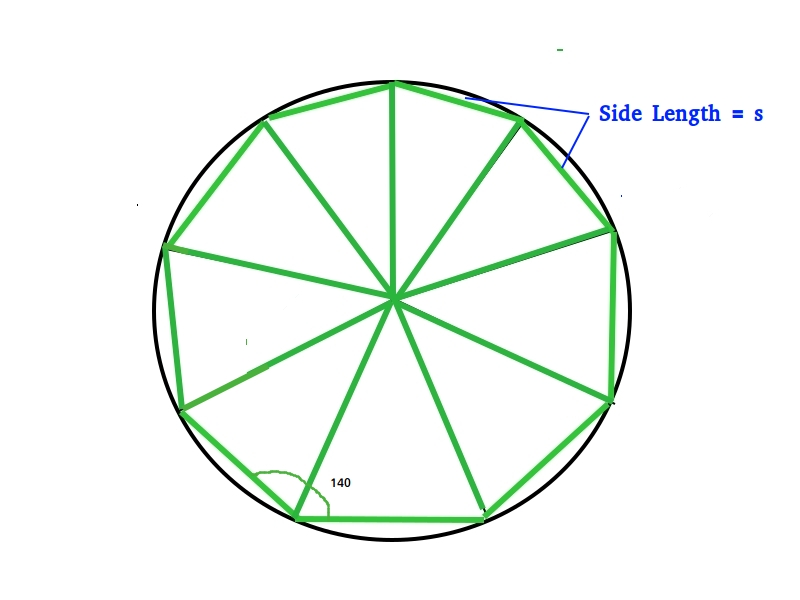# Program to calculate area of Enneagon

Enneagon is a polygon with 9 sides and 9 internal angles. Enneagon is also known as Nonagon. A regular nonagon has an internal angle of 140 degrees each. Sum of interior angles of the nonagon is 1260 degree.
The center of the circumcircle is also taken as the center of the regular Nonagon.
The line segment drawn the perpendicular to a side of the Nonagon is called the Apothem and is represented by ‘a’.``` Area &approx; 6.1818 * s * s
where s is side length.
```

Examples:

```Input : 6
Output :Area of Regular Nonagon = 222.5448

Input : 8
Output :Area of Regular Nonagon = 395.6352
```

## C++

 `// CPP program to find area of a Enneagon ` `#include ` `#include ` `#include ` `using` `namespace` `std; ` ` `  `// Function to calculate area of nonagon ` `double` `Nonagon_Area(``double` `s) {  ` `  ``return` `(6.1818 * s * s);  ` `} ` ` `  `// driver function ` `int` `main() { ` `  ``double` `s = 6; ``// Length of a side ` `  ``cout << ``"Area of Regular Nonagon = "` `<< std::setprecision(7) ` `       ``<< Nonagon_Area(s); ` `  ``return` `0; ` `} `

## Java

 `// Java program to find area of a Enneagon ` `class` `Nonagon { ` ` `  `  ``// Function for calculating the area of the nonagon ` `  ``public` `static` `double` `Nonagon_Area(``double` `s) {  ` `    ``return` `((``6.1818` `* (s * s)));  ` `  ``} ` ` `  `  ``// driver code ` `  ``public` `static` `void` `main(String[] args) { ` `    ``double` `s = ``6``; ``// Length of a side ` `    ``System.out.print(``"Area of Regular Nonagon = "` `+ Nonagon_Area(s)); ` `  ``} ` `} `

## Python

 `# python program to find area of a Enneagon ` `length ``=` `6` `Nonagon_area ``=` `6.1818` `*` `(length ``*``*` `2``)  ` `print``(``"Area of regular Nonagon is = "``, Nonagon_area) `

## C#

 `// C# program to find area of a Hexagon ` `using` `System; ` ` `  `class` `Nonagon { ` ` `  `    ``// Function for calculating  ` `    ``// the area of the nonagon ` `    ``public` `static` `double` `Nonagon_Area(``double` `s) ` `    ``{ ` `        ``return` `((6.1818 * (s * s))); ` `    ``} ` ` `  `    ``// driver code ` `    ``public` `static` `void` `Main() ` `    ``{    ` `        ``// Length of a side ` `        ``double` `s = 6;  ` `        ``Console.WriteLine(``"Area of Regular Nonagon = "` `+ ` `                                       ``Nonagon_Area(s)); ` `    ``} ` `} ` ` `  `// This article is contributed by vt_m `

## PHP

 ` `

Output:

```Area of Regular Nonagon = 222.5448
```

My Personal Notes arrow_drop_upCheck out this Author's contributed articles.

If you like GeeksforGeeks and would like to contribute, you can also write an article using contribute.geeksforgeeks.org or mail your article to contribute@geeksforgeeks.org. See your article appearing on the GeeksforGeeks main page and help other Geeks.

Please Improve this article if you find anything incorrect by clicking on the "Improve Article" button below.

Improved By : vt_m

Article Tags :
Practice Tags :

Be the First to upvote.

Please write to us at contribute@geeksforgeeks.org to report any issue with the above content.